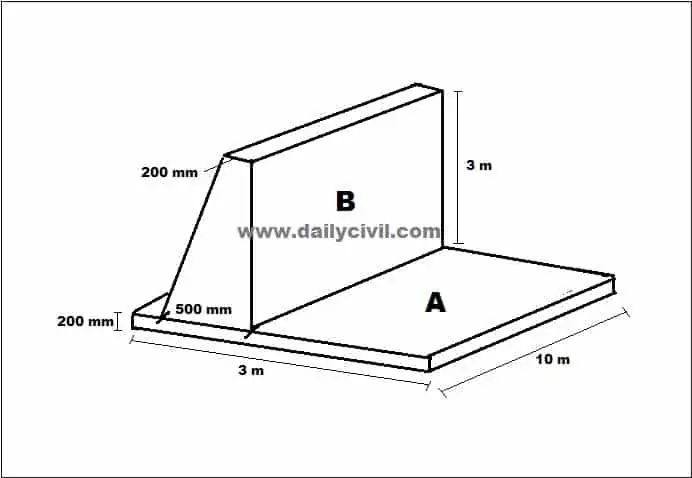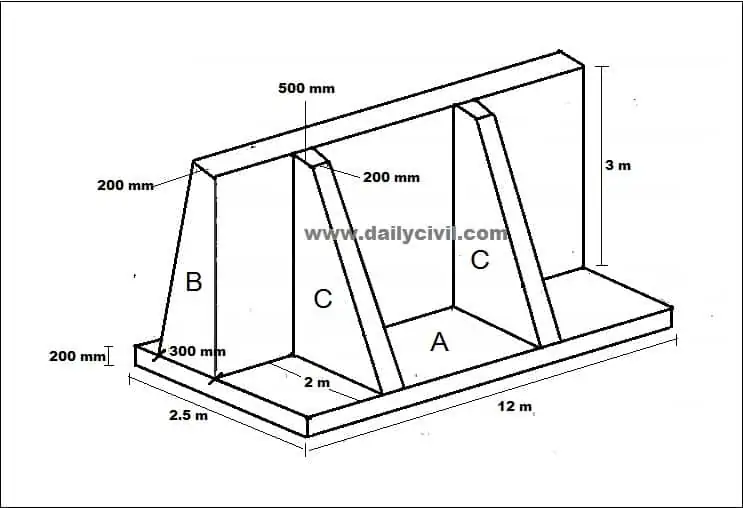# How To Calculate Concrete Volume For Retaining Wall

## How To Calculate Concrete Volume For Retaining Wall:

Retaining wall is a masonry wall constructed to resist the pressure of liquid, earth filling, sand or other granular material filled behind it.

In this article, I will discuss how to calculate the concrete volume for retaining wall. Let’s get started.

Example 1:To calculate the volume of retaining wall we need to calculate the volume of retaining wall.

Here I have divided the retaining wall into two parts, part A is the base slab and part B is the stem of retaining wall.

So Volume of retaining wall = Volume of base slab + Volume of stem.

Volume of base slab = l x b x h = 10 x 3 x 0.2 = 6 m³

The stem is a trapezoid.

So Volume of stem = [{(a + b)/2} x h] x l

= [{(0.5 + 0.2)/2} x 3] x 10

= 21 m³

The total volume of retaining wall = 6 + 21 = 27 m³

So the volume of concrete for the retaining wall = 27 m³

Let’s take another example:

Example 2:Here I have divided the retaining wall into three parts, part A is the base slab, part B is the stem and part C is the counterfort of the retaining wall.

The volume of retaining wall = Volume of base slab + Volume of stem + Volume of counterfort

= Volume of A + Volume of B + Volume of C

Volume of A = l x b x h = 12 x 2.5 x 0.2 = 6  m³

Part B is a trapezooid.

So Volume of B = [{(a + b)/2} x h] x l = [{(0.2 + 0.3)/2} x 3] x 12

= 9 m³

As part C is also a trapezoid

So

Volume of C = [{(a + b)/2} x h] x l = [{(0.5 + 2)/2} x 3] x 0.2 = 0.75 m³

In the above retaining wall there is 2 counterfort so

Volume of C = 0.75 x 2 = 1.5 m³

The total volume of retaining wall = 6 + 9 + 1.5 = 16.5 m³.

### 8 thoughts on “How To Calculate Concrete Volume For Retaining Wall”

1. It is such as helpful page thanking for your

2. Very helpful for civil engineering.

3. Very good explanation

4. very grate explanations

5. V= L(a+b/2)h yeh ho sakata hai